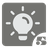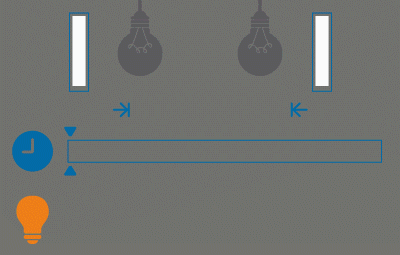Lightbulb Operating
Simple+
RU English

Since you are here, it means that you’ve already solved 4 missions of the series. Your function can already adopt more than one light bulb in the date array to determine if the room is lit or not. And with the second and third elements the period we want to observe can be defined.

In the 5th mission, a fourth argument is added - the operating time of the light bulbs. By analogy with the previous missions - if it’s not passed, then the bulb works indefinitely.

The operating time argument is passed as a timedelta object. It shows how long the light bulb can work when it’s on. It has no cooling, which means that if our bulb can work for only one hour, then it can work for 30 minutes now and 30 minutes next year. After that it will turn itself off and will no longer respond to the button.

We still need to calculate how long the room has been lit.Input: Four arguments and only the first one is required. The first one (els) is a list of datetime objects (instead of datetime object there can be a tuple of two datetime and int), the second (start_watching) and the third ones (end_watching) is the datetime objects. The forth argument (operating) - timedelta object - how long the lamp can work.

Output: A number of seconds as an integer.

Example:

```sum_light([
datetime(2015, 1, 12, 10, 0, 0),
datetime(2015, 1, 12, 10, 0, 30),
(datetime(2015, 1, 12, 10, 0, 30), 2),
(datetime(2015, 1, 12, 10, 1, 0), 2),
], operating=timedelta(seconds=20)) == 40

sum_light([
(datetime(2015, 1, 12, 10, 0, 10), 3),
datetime(2015, 1, 12, 10, 0, 20),
(datetime(2015, 1, 12, 10, 0, 30), 3),
(datetime(2015, 1, 12, 10, 0, 30), 2),
datetime(2015, 1, 12, 10, 0, 40),
(datetime(2015, 1, 12, 10, 0, 50), 2),
(datetime(2015, 1, 12, 10, 1, 20), 2),
(datetime(2015, 1, 12, 10, 1, 40), 2),
], start_watching=datetime(2015, 1, 12, 10, 0, 20), operating=timedelta(seconds=100)) == 50

sum_light([
(datetime(2015, 1, 12, 10, 0, 10), 3),
datetime(2015, 1, 12, 10, 0, 20),
(datetime(2015, 1, 12, 10, 0, 30), 3),
(datetime(2015, 1, 12, 10, 0, 30), 2),
],
start_watching=datetime(2015, 1, 12, 10, 0, 10),
end_watching=datetime(2015, 1, 12, 10, 0, 30),
operating=timedelta(seconds=5)) == 10
```

Precondition:

• The array of pressing the button is always sorted in ascending order.
• The array of pressing the button has no repeated elements.
• The minimum possible date is 1970-01-01
• The maximum possible date is 9999-12-31